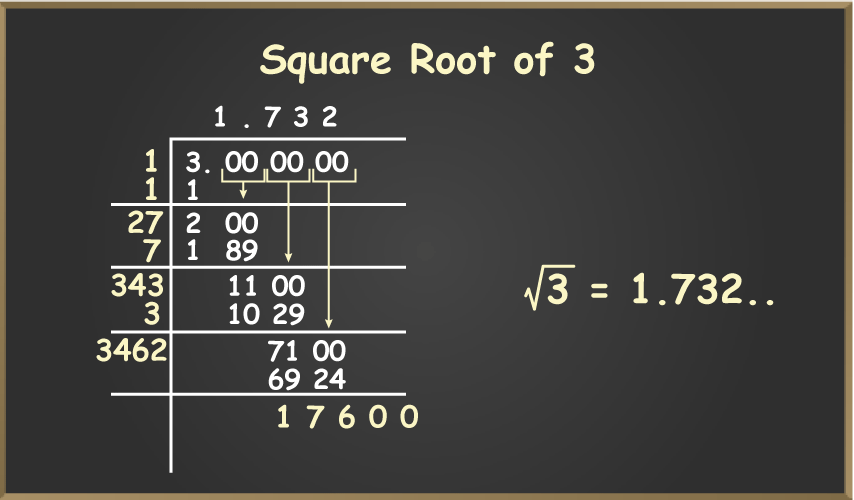GFG App
Open AppBrowser
Continue

Square root of 3 is a positive real number which will give the number 3 when multiplied by itself. The square root of 3 is an irrational number as it is a non-terminating and non-repeating decimal. Square root of 3 is denoted as √3 or 31/2. As the square of 3 is an irrational number so its exact decimal value is never calculated.

Value of square root of 3, √3 =1.732 (truncated)

## What is the Square Root of 3?

Square root of a number is a number that when multiplied by itself, results in the original number.

Example: Square root of 16 is 4, as 4×4 = 16 so √16 = 4

Square root of 3 or simply called root 3 is an irrational number which by multiplying by itself results in the number 3. We can give the approximate value of root 3 as,

• Decimal form: 1.732
• Exponent form: 31/2

## Root 3 Value in Maths

In mathematics, finding the square root of any number is very important, the square root of the perfect square can easily be calculated. For imperfect squares finding a square root is difficult and is generally calculated using the long division method.

Root of 3 is expressed as √3 it is an irrational number so its decimal value can never be computed. It is represented in the radical form as (3)½. Square root of 3 rounded up to 7 decimal places is 1.7320508.

Square Root of 3 = 1.7320508075688772…

## Is the Square Root of 3 Rational or Irrational?

Square root 3 is a non-terminating and non-repeating decimal and all non-terminating and non-repeating decimal comes under irrational number. So it is safe to say that √3 is an irrational number.

The decimal expansion of the square root of 3 is,

√3 = 1.7320508075688772…

As it is non-repeating and non-terminating we can surely say √3 is irrational.

## How to Find the Square Root of 3?

It is always easier to compute the square root of perfect squares but to compute the square root of a non-perfect square, we need to perform the long division method.

To compute the square root of 3, we need to follow the steps given below:

Step 1: Write 3 as 3.000000 (where zeros are always kept in form of pairs) to make it easier to divide.

Step 2: Now look for the perfect square less than 3, i.e., 1, and divide the number with it.

Step 3: Now the quotient is 1 and the remainder is 2. We will place a decimal in the quotient and bring down the pair of zeros for further division.

Step 4: Now the next dividend is 200 add the next divisor will be 2X (Quotient 1 is added to the previous divisor 1 and X is at tenth place), such that the multiplication of the number 2X and X should be less than 200. This will give the next value 27 as the new divisor.

Step 5: Now 7 is added to the quotient after a decimal, so the value of the quotient will be 1.7 and the new divisor will be obtained by adding 7 to 27, therefore 34Y, where Y is at the hundredth place.  Using the previous steps we can go on and will obtain the square root of 3 in the quotient.Now. bring down the next pair of zeros and repeat steps 4th and 5th. This can be done for infinite steps as the exact value of the square root of 2 goes up to infinite decimal places. We can compute the result up to 4 decimal places as that can be used for approx. value of the square root.

## Properties of Square Root of a Number

Various properties of the Square Root of a Number are discussed below,

• Perfect square numbers have perfect square roots.
• Square root of an even perfect square is even.
• Square root of an odd perfect square is odd.
• Square root of a negative number is a complex number.

## Table of Square Root

Square roots of various numbers are widely used in mathematics. The table given below gives us the square root of various numbers which are used commonly.

Also, Check

## FAQs on the Square Root of 3

### Question 1: What is the value of the Square Root of 3?

The approximate value of square root of 3 is 1.73205.

### Question 2: Why is the Square Root of 3 an Irrational Number?

The value of Square Root of 3 is a non-repeating and non-terminating decimal so it is an irrational number.

### Question 3: Is the number 3 a Perfect Square?

3 is a prime number and prime numbers have no factors other than 1 and themself. Thus, we can say that 3 is not a perfect square.

### Question 4: How can we find the value of √3?

The value of √3 can be found using, the long division method. As, 3 is not a perfect square so its exact value is never found.